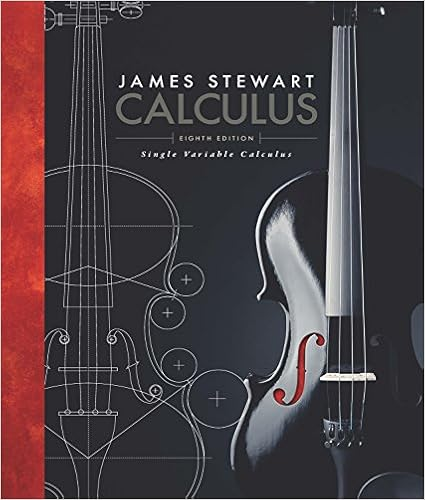Assignment - Relations and Functions - Assignment Relations...

• Homework Help
• 4
• 91% (11) 10 out of 11 people found this document helpful

This preview shows page 1 - 3 out of 4 pages.

We have textbook solutions for you!
The document you are viewing contains questions related to this textbook.The document you are viewing contains questions related to this textbook.
Chapter 6 / Exercise 1
Single Variable Calculus
StewartExpert Verified
Assignment: Relations and Functions Follow the directions to solve all the parts of each problem. Show all work leading to your answer. 1. There are many ways to describe relations and function. Consider the relation shown as a set of ordered pairs: {(2,3), ( −1,5), (4,3), (0,5), (3, −2)} a. Describe this relation in three different ways: Make a mapping diagram. List the domain and range in set notation.
b. Does this relation represent a function? How do you know?
We have textbook solutions for you!
The document you are viewing contains questions related to this textbook.The document you are viewing contains questions related to this textbook.
Chapter 6 / Exercise 1
Single Variable Calculus
StewartExpert Verified
2. Create a relation of 5-8 ordered pairs similar to those given to you in Problem 1. Your relation should be written using proper set notation as shown in the example problem.
Describe this new relation in three different ways: Make a mapping diagram. List the domain and range in set notation.
•••